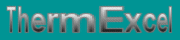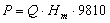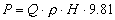Mechanical energy provided to the fluid (Closed loop), mechanical energy in hydrostatic load (Fluid on open circuit) - degraded Energy (Power at the shaft of the pump) Pumps head, Pumps horsepower, Pumps Net Positive Suction Head.
French site
| Home | Thematic | Tables | Programs | Library | Download | Links | Contact | Forum |--

Pumps
 Thematic Quotation Margin Hydraulic Linear pressure loss Local pressure loss Compressible fluids No circular duct Thermal Thermal fluid flow Pipe sizing steam Control valves Orifice plate Pumps Ventilators Gas Fuel gasEnergy provided by the pump

In the hydraulic field, the load of a pump is expressed in theory in height of water.

The absorptive energy by the pump breaks up:

Mechanical energy provided to the fluid (Closed loop)

It is the hydraulic power communicated to the liquid of its passage through the pump.

This mechanical power is given by the following formula:With:

• P = Power transmitted to the fluid by the pump in Watt.
• Q = Flow in m3/s.
• Hm = Energy or pressure loss of the hydraulic network expressed in m.

Mechanical energy in hydrostatic load (fluid on open circuit)With:

• P = Power transmitted to the fluid by the pump in Watt.
• Q = Flow in m3/s.
• p = Density of the liquid in kg/m3.
• H = Piezometric height in meter of water.
• 9.81 = Average Intensity of gravity.

Mechanical energy provided to the liquid (For example, distribution network of drinking water):+With:

• P = Power transmitted to the fluid by the pump in Watt.
• Q = Flow in m3/s.
• p = density of the liquid in kg/m3.
• Hm = Hydraulic pressure loss network expressed in m.
• H = Hydraulic load in meter of water.
• 9.81 = Average Intensity of gravity.

Degraded energy expressed by the output of the pump (Power at the shaft of the pump)

It is the power measured on the shaft of the pump.

Mechanical energy necessary to a pump is always higher than the energy transmitted to the fluid, following various frictions of the bodies of rotation.With:

• Pmec = Mechanical power necessary to the pump.
• Pfl = Power transmitted to the fluid.
• Rv = Output of the ventilator.
• Rt = Output of the transmission.

In the centrifugal pumps, the essence of degraded energy overheats is the pumped liquid.

In the reciprocating pumps, the essence of degraded energy is in the mechanical drives and is not communicated to the liquid.

The outputs generally allowed are:

• Piston pumps = 0.6 à 0.7
• Centrifugal pumps = 0.4 à 0.8

Motorization

At the time of the choice of the engine, it is the absorptive power by the pump which determines the power delivered by the engine and thus also the absorptive power at the network. It is necessary thus to take guard so that the engine has a sufficient power to satisfy all the situations of operation of the installation.

Let us take the case of a pump having an absorptive power of 8.5 kW. The engine will provide these 8.5 kW, independently owing to the fact that it is conceived for 7 kW or 10 kW. An engine of 7 kW, having to function at 40°C, would thus always be overloaded of 21.5 %.

The direct consequence of an overload of the engine is an increase in the temperature of winding. A overtaking of the limiting temperature of 8-10°C, decreases the lifespan of the insulation of approximately half. Overtaking beyond of 20°C mean shortening of 75 %.

The engines of standard construction are planned for a use at maximum ambient temperature of 40°C (and a maximum altitude of the site of 1 000 m). Any variation requires a correction of the nominal output.

Last update: